Google Meet team, what were you thinking?
‘Ctrl+D’ as the key combo to mute/unmute your microphone? 😮
(It’s also the combo for ‘Add Bookmark’, and depending on browser context Meet doesn’t always manage to trap the input. And it’s clunky.)

I missed having a push-to-talk key, so I added my own. 🎆📻

Now in my video calls, I just hold down space to un-mute the mic, and let go to re-mute. Much better.
(and it should work for any language, and not interfere with any existing Meet functionality including chat)

• Available as a Greasemonkey script: Google Meet Hangouts – PTT.js
• Or as a bookmarklet:
javascript:(()=>{var e=void 0;const t=t=>({key:d,repeat:n,target:o})=>{e&&e.isConnected||(e=document.querySelector('[data-is-muted][role="button"]')),e&&" "===d&&!n&&!("value"in o)&&e.dataset.isMuted===String(t)&&e.click()};document.body.addEventListener("keydown",t(!0)),document.body.addEventListener("keyup",t(!1))})();

In my previous post, I simulated some mechanical logic gates using a novel 3-bar linkage.
The dimensions of the linkage are critical to achieving correct operation;

Inputs $$\{ false\ false \}$$, $$\{ false\ true \}$$, $$\{ true\ false \}$$ must all produce the same output position.

For the simulations, an empirical approach was used. (fiddle with the values until it looks right)

This time, I’m going to attempt to find the exact values to use for a given stroke, separation distance and output position.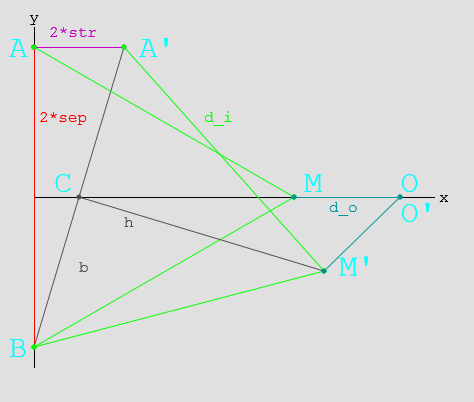Here, you can see the linkage layout for $$\{ false\ false \}$$ and $$\{ true\ false \}$$

# Mathematics

Ahead lurks tough mathematics (which may be unsuitable for liberal-arts majors)
If it’s hard to read, then sorry! It was hard to write!

## Parameters

• $$2 sep$$ Separation between inputs
• $$2 str$$ Stroke length $$false \to true$$
• $$d_i$$ Input link length
• $$d_o$$ Output link length

## Geometry

• $$A(x_a, y_a) = A(0, sep)$$ is the input position when $$A_{state}=false$$
• $$A'(x_a’, y_a’) = A'(2*str, sep)$$ is the input position when $$A_{state}=true$$
• $$B(x_b, y_b) = B(0,-sep)$$ is the input position (The system is symmetric, $$B’$$ does not need to be tested.)
• $$M(x_m, y_m)$$ is the middle position when $$A_{state}=false$$
• $$M'(x_m’, y_m’)$$ is the middle position when $$A_{state}=true$$
• $$O(x_o, y_o)$$ is the output position. ($$O \equiv O’$$ for the gate to function correctly.

## Solving

### Finding $$M$$:

$$M(x_m, y_m) = M( \sqrt{d_i^2 – sep^2}, 0)\\$$

### Finding $$O$$:

\begin{align} O(x_o, y_o) &= O( x_m + d_o, 0 )\\ & = O( \sqrt{d_i^2 – sep^2} + d_o, 0) \end{align}

### Finding $$M’$$:

• $$C'(x_c’, y_c’)$$ is the midpoint of $$BA’$$
• $$b$$ is the length of the line $$BC’$$
• $$h$$ is the length of the line $$C’M’$$

\begin{align} x_c’ &= (x_a’ + x_b)/2 \\ &= str\\ y_c’ &= (y_a’ + y_b)/2 \\ &= 0\\ b &= \sqrt{ sep^2 + str^2 }\\ h &= \sqrt{ d_i^2 – b^2 } && Because BC’ \bot C’M’\\ &= \sqrt{ d_i^2 – sep^2 – str^2 }\\ \end{align}\\

Using this, we can calculate M’:
\begin{align} \frac{h}{b} &= \frac{\sqrt{ d_i^2 – sep^2 – str^2 }}{\sqrt{ sep^2 + str^2 }} \\ &= \sqrt{\frac{ d_i^2 – sep^2 – str^2 }{ sep^2 + str^2 }} \\ &= \sqrt{\frac{d_i^2}{sep^2 + str^2} – 1}\\ \\ x_m’ &= x_c’\ – ( y_c’ – y_b)\frac{h}{b}\\ &= str – \sqrt{\frac{d_i^2}{sep^2 + str^2} – 1} \\ y_m’ &= y_c’ + ( x_c’ – x_b)\frac{h}{b}\\ &= str \sqrt{\frac{d_i^2}{sep^2 + str^2} – 1} \end{align}\\

### Finding $$O’$$:

\begin{align} x_o’ &= x_m’ + \sqrt{ d_o^2 – y_m’^2 }\\ &= ( str – \sqrt{\frac{ d_i^2 }{sep^2 + str^2} – 1}) + \sqrt{ d_o^2 – ( str \sqrt{\frac{d_i^2}{sep^2 + str^2} – 1} )^2 }\\ &= str – \sqrt{\frac{ d_i^2 }{sep^2 + str^2} – 1} + \sqrt{ d_o^2 – str^2 (\frac{d_i^2}{sep^2 + str^2} – 1) }\\ y_o’ &= 0 \end{align} \\

## And then:

Now that $$O$$ and $$O’$$ are found, and we know $$O \equiv O’$$…

### Finding the $$x_o’$$ equation in terms of $$d_o^2$$:

\begin{align} x_o’ &= x_m’ + \sqrt{ d_o^2 – y_m’^2 }\\ d_o^2 – y_m’^2 &= (x_o’ – x_m’)^2 \\ d_o^2 – str^2 (\frac{d_i^2}{sep^2 + str^2} – 1) &= (x_o’ – str + \sqrt{\frac{d_i^2}{sep^2 + str^2} – 1})^2 \\ d_o^2 &= \left( x_o’ – str + \sqrt{\frac{d_i^2}{sep^2 + str^2} – 1} \right)^2 + str^2 (\frac{d_i^2}{sep^2 + str^2} – 1) \\ \end{align}

### Substituting back into the $$x_o$$ equation:

\begin{align} x_o &= \sqrt{d_i^2 – sep^2} + d_o \\ x_o – \sqrt{d_i^2 – sep^2} &= d_o \\ ( x_o – \sqrt{d_i^2 – sep^2} )^2 &= d_o^2 \\ ( x_o – \sqrt{d_i^2 – sep^2} )^2 &= \left( x_o’ – str + \sqrt{\frac{d_i^2}{sep^2 + str^2} – 1} \right)^2 + str^2 (\frac{d_i^2}{sep^2 + str^2} – 1) \end{align}
Ugly, but workable?

# Example:

• $$sep = 50$$ Separation between inputs $$100 mm$$
• $$str = 20$$ Stroke length $$false \to true: 40 mm$$
• $$d_i = ?$$ Input link length
• $$d_o = ?$$ Output link length
• $$x_o = x_o’ = 70$$ Desired output position

\begin{align} ( x_o – \sqrt{d_i^2 – sep^2} )^2 &= \left( x_o’ – str + \sqrt{\frac{d_i^2}{sep^2 + str^2} – 1} \right)^2 + str^2 (\frac{d_i^2}{sep^2 + str^2} – 1) \\ ( 70 – \sqrt{d_i^2 – 50^2} )^2 &= \left( 70 – 20 + \sqrt{\frac{d_i^2}{50^2 + 20^2} – 1} \right)^2 + 20^2 (\frac{d_i^2}{50^2 + 20^2} – 1) \\ \end{align} \\
Pushed through Wolfram Alpha…
\begin{align} \\ d_i &= \sqrt{2900} \\ &= 53.852\\ \text{and}\\ d_o &= x_o – \sqrt{d_i^2 – sep^2} \\ &= 70 – \sqrt{ 2900 – 2500 } \\ &= 50 \end{align}

# Summary:

Phew!

Thusly, one can calculate the input and output linkage lengths for any logic gate.
These dimensions will work for an OR gate too, as the linkages are just reversed.

It’s irksome that I couldn’t work out a symbolic solution for the last equations, especially since $$d_i$$ in the example came out to such a neat value.

I might revisit this further in the future, and it’ll definitely come in handy when building something with these gates.

# Springless Reversible Mechanical Logic

Logic gates! The building blocks of computing as we know it. What if we ditch the electrons?

I want some mechanical logic for an upcoming project, so I did some research…

# Research

There are several examples of mechanical logic out there on the internet:

Keshav Saharia’s Lego LogicPush-pull, needs a spring return.
Randomwraith’s Lego LogicPush-pull with internal rotating elements, reversible.
Mechalogic’s Logic ElementsPush-pull, AND & NOT reversible, OR needs a spring return.
Xiaoji Chen’s Linkage ComputerRotating logic, reversible, some mechanical issues with parallelogram weakness.
Spillerrec’s Lego Logic GatesPush-pull, very compact, needs a spring return.
He identifies the problem with needing springs, but doesn’t have a fix. Mentions how AND/OR gates can be reversed to become OR/AND gates.
Zeroumus’s AND GatePush-pull, needs a spring return.
KNEX XOR GatePush-pull, needs a spring return.
Carolin Liebl and Lisa Hopf’s NAND GatePush-pull, reversible, complex.

There are many more ideas out there, even water pipe logic, and of course the early mechanical computers Digi-Comp I and Digi-Comp II.

For my project, I have the following requirements;

• Push-pull logic
• Output movement range must equal input movement range
• No springs/elastic bands, and no forces for inputs to overcome
• No gears!
• Easily chain-able, and simple enough to implement a score of them.

This disqualifies most of the designs I found, particularly the ‘no springs’ requirement.

# AND Gate

Mechalogic’s AND gate design uses an elastic band, but doesn’t appear to need it, so it serves as a good starting point: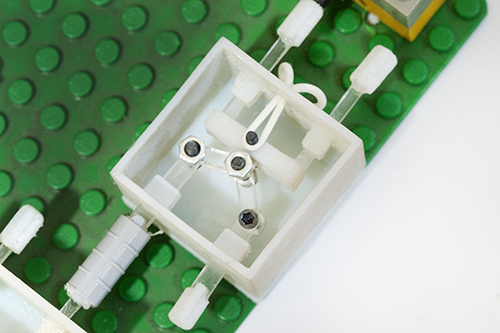It’s time consuming to tweak parameters in the physical world, so I wrote a small simulation: (click to see in action)Success! Meets all the requirements, and only needs three parts.

The geometry is important – the ratio will determine the output’s position when only one of the inputs is asserted, according to;

• distance between the inputs (red),
• length of the input links (green),
• length of the output link (teal)
• input displacement

Now, can an OR gate be designed with the same success?

# OR Gate

Most OR gates look like this; the output (on the left of this picture) has a flat plate, and either input will push the flat forward. Unfortunately the design needs that elastic band to return the output to zero.Inspired by some earlier attempts to couple an AND and a backwards OR gate together, I tried flipping the AND gate around; (click to see in action)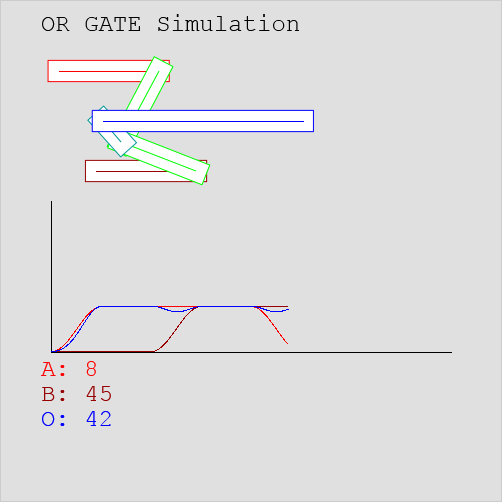Success! Oddly enough, an OR gate is just an AND gate connected up backwards.
Even the linkage lengths are the same!
Best of all, the OR gate is reversible like the AND gate – no springs and no force required.

# AND Then?

As the graphs show, output displacement equals input displacement, so these designs should be chainable. Let’s have a look; (click to see in action)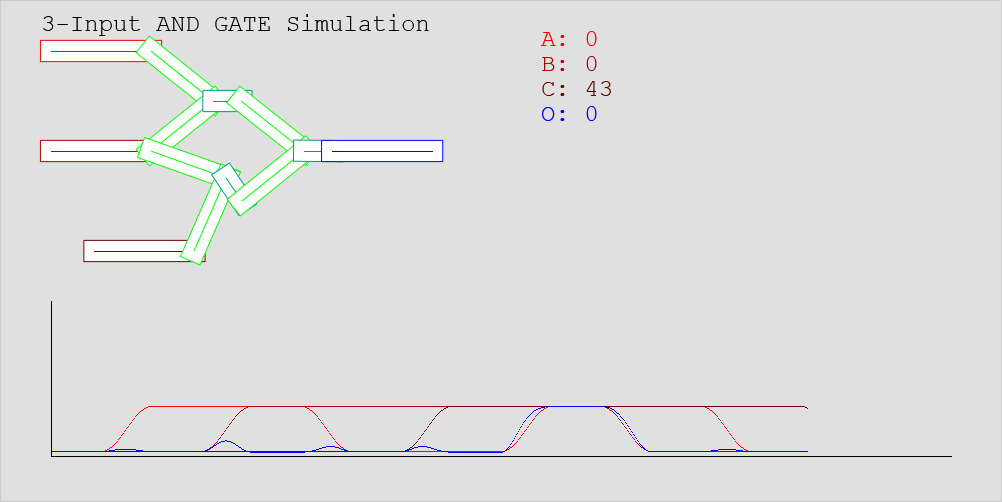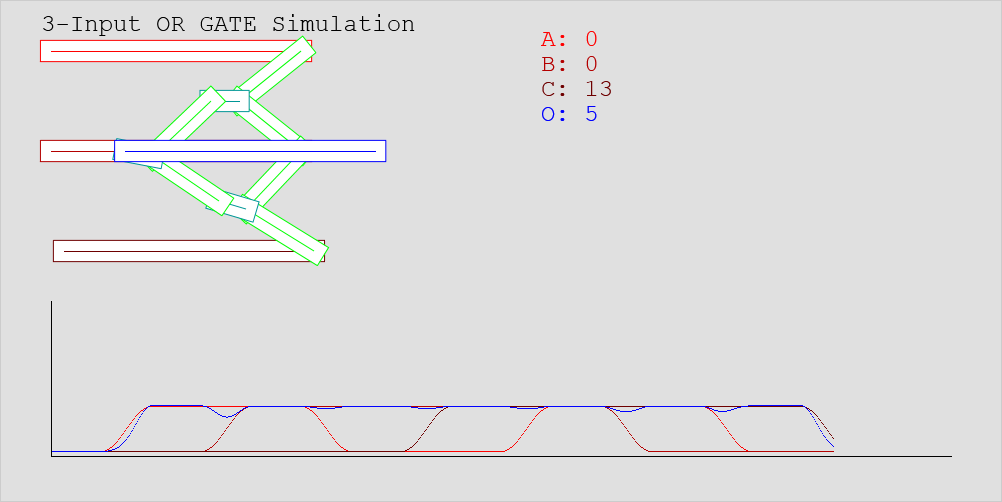Success!

Now I just have to build something complex with them…

All the simulations are viewable here.
The code they’re written with is available on GitHub;
https://github.com/MrTrick/logic_gates

# Javascript: Self-replacing Promises

My application has several resources that need to be loaded just once, and used often afterwards.

Here is a pattern that I’m finding quite useful lately:

/**
* Retrieve the resource once only.
* If the resource hasn't been loaded yet,
*   return a deferred that resolves to the resource.
* If the resource has been loaded,
*   return the resource directly.
*/
App.getResource = function() {
//Return the resource or the deferred if it's set already
if (App._resource)
return App._resource;
//Otherwise build, set and return a deferred that
else
return App._resource = $.getJSON("resource/url").then( function(data) { console.debug("Loaded resource"); return App._resource = data; } ); }; • The first time the function is called, it fetches the resource and returns a deferred object. • When the promise resolves, it passes the resource to handlers. • If called again before the promise resolves, it returns the same promise. (won’t fetch twice) • After the promise has resolved, the function instead returns the resource directly. • If the resource can’t be loaded, the promise fails instead, and is not replaced. To use directly; $.when(App.getResource()).done(function(resource) {
//Use the parameter from the promise
resource.doStuff();
});

The $.when call transparently converts a non-promise into a resolved promise with that value. The snippet behaves the same whether App.getResource() has been previously loaded or not. So why replace the promise at all? Sometimes you need to be able to treat the resource like a synchronous value, such as within a library or a function that is called synchronously. //Here's a class with a synchronously-called function var Model = Backbone.Model.extend({ ... validate: function(attrs) { if (App.getResource().exists(attrs[id])) { return "Model with that id already exists"; } else { return null; } } }); var model = new Model(); //Wait for everything necessary to load first.$.when(App.getResource(), model.fetch(), ...).done(
function() {
if (model.isValid()) doFoo();
else doBar();
});

This code waits for App.getResource() to resolve, as well as for any other needed promises. Afterwards, App.getResource() can just be treated like a synchronous function.

This pattern enables flexible and transparent use of remote resources, in both synchronous and asynchronous ways.

The App.getResource() function is also quite similar to a Lazy Promise, as discussed by James Coglan.

# Atmel Studio: Clearing debugWIRE

debugWIRE is a fantastic bit of technology in many recent AVR chips, allowing you to set breakpoints, step through your code, and a bunch of other useful features. All you need is a programmer that supports it – the AVR Dragon for instance.

In the Atmel Studio project, simply set the Debugger/Programmer Interface to debugWIRE.

The only fly in the ointment is that for extremely low-power applications, having the DWEN fuse and thus debugWIRE enabled will prevent the chip from fully sleeping, meaning your device is much more thirsty than expected.

So you set the Debugger/Programmer Interface back to ISP, and try to program your device again; Failure! In fact; CRYPTIC ERROR MESSAGE!

TO CLEAR debugWIRE FROM A CHIP WITHIN Atmel Studio 6:
Start a debug session on the chip, then
Go to the “Debug” menu and select “Disable debugWIRE and close”.

Now you can access the chip using ordinary ISP, and low-power devices will be more frugal.

This may seem an obvious thing, but there are a number of “solutions” floating around, including people advocating hooking HVPP programmers into devices simply to clear DWEN!

# More Javascript Templates Using ‘src’

There was some concern about my previous solution, that it loads templates synchronously and serially. An application should have templates inlined before it goes to production anyway.

Fair point though, there is a better way.

## Better Definition

Firstly; Marionette.js can transparently lazy-load and cache DOM-stored templates;

//What I had been doing
var MyView1 = Marionette.ItemView.extend({
template: _.template( $("#template_myview1").html() ) }); //What I should have been doing var MyView2 = Marionette.ItemView.extend({ template: "#template_myview2" }); The difference between the two is significant. • MyView1 will access the DOM as soon as the class is defined, and build the template immediately. • MyView2 will access the DOM only when a MyView2 instance is constructed, but only builds the template once. This means the templates are only built at the time they are first used. The template blocks don’t need to be accessible at the time the classes are defined. The app only needs to build the template functions the first time they are used. Using the MyView2 style will give much faster start-up times for larger applications. (Marionette.TemplateCache can be used stand-alone, or you can fairly easily create your own caching DOM template loader) ## Better Loading Now, the template blocks won’t be referenced until they are used. We can load them asynchronously now, but we still don’t want to start the app until the templates have been fetched.$.ready(…) won’t wait for the templates, but there’s a fairly easy workaround;

//A list of promises that need to resolve before starting

var $templates =$('script[type="text/template"]');
$templates.each(function() { var src =$(this).attr("src");
if (src) {
$.ajax(src, {context:this}). done(function(data) {$(this).html(data);
})
);
}
});

//Wait for the DOM to be ready
loaded.push($.ready); //Initialise after everything is loaded$.when.apply($,loaded).done(function() { //Start application }); There! With that in place; • Templates are loaded into script blocks as you’d expect, asynchronously and in parallel. • The application starts after the DOM and the templates are loaded. • For production, the templates are inlined. Without any code changes, the application will transparently start as soon as$.ready is completed.

# Background

John Resig was the first to document a technique for storing templates in <script> tags;

<script type="text/html" id="item_template">
<li><b>{{ name }}</b> - {{ description }}</li>
</script>

This works well for several reasons;

1. Using the unknown type “text/html” means that the browser ignores it.
2. Giving the tag an id allows us to easily grab its contents;
//Build a new item, and add it to the list
//Using JQuery, Underscore.js
var contents = _.template(
$("#item_template").html(), {name: "Foo", description:"Sir Foo the Second"} );$list.append( $(contents) ); Today there are many libraries available for retrieving and building templates in this way, such as Mustache.js and ICanHaz.js, and more that integrate support. # Problem One of the only problems with this technique, is that the scripts must be declared inline. <!-- Works! --> <script type="text/html" id="item_template"> <li><b>{{ name }}</b> - {{ description }}</li> </script> <!-- Doesn't work. --> <script type="text/html" id="item_template2" src="templates/item2.html"></script> If the browser doesn’t understand the type, it won’t load the source. Whatever the reason, the template text is never added to the DOM. Inlining template contents is detrimental to readability, maintainability, and traceability. Some libraries offer asynchronous methods for loading templates. (require.js among them) Some have issues with complexity and performance. It feels natural to define and compile the template functions at class definition time. Other solutions may add unnecessary layers of indirection. # Solution I’ll preface this by saying “Okay, maybe this won’t work for you”, but so far it’s working well, transparently, and fast. And when you go to production, a decent deployment optimisation process will inline the templates anyway. What if the src attributes were read properly? <script type="text/html" id="template_widgets" src="templates/widgets.html"></script> <script type="text/html" id="template_widgets_item src="templates/widgets_item.html"></script> ... ... <script type="text/javascript"> //Synchronously load the templates var$templates = $('script[type="text/html"]');$templates.each(function() {
//Only get non-inline templates
//No need to change code for production.
if ($(this).attr("src")) {$.ajax(
$(this).attr("src"), { async:false, context:this, success:function(data) {$(this).html(data); }
}
);
}
});
//From this point onwards (in the same script, in the
//following scripts) all the "text/html" script tags
//will be loaded as if they were inline.
</script>

Just insert this code after all your template tags, and before you build your template functions, and you’ll be able to treat them as if they were inline all along.

Easy.* 🙂

*There are some downsides to loading template serially and synchronously. I’ve written a follow-up article that discusses a technically better solution.

# Multiple Pages with Backbone.js and Bootstrap

Backbone.js has routes. Hooray, now ‘#here’ can go here, ‘#there’ can go there, and so on! That’s the logic side sorted out.

In many cases, 1 route <=> 1 ‘page’ of content.

How do we do that?

The Bootstrap library has a tabs component that implements a couple of very useful things:

• Provides a link between a menu and a set of ‘pages’
• Easy to trigger page changes

We still need to add two small things to connect any router to a set of pages:

• When a route is triggered, activate a matching tab.
• When a tab is activated, trigger any matching route.

All it needs is the following code:

//On click, show the page, allow to route.
$('#tab_demo_menu a').on('click', function (e) {$(this).tab('show');
}
//On route, trigger the 'click'
Backbone.history.on('route', function(router, event) {
});